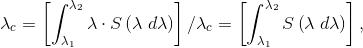Jul 5, 2018

[4.7.2] λc

The wavelength calculated as the weighted mean of a spectral function according to:where:

The wavelength calculated as the weighted mean of a spectral function according to: λ is the wavelength, S(λ) is the spectral power distribution function, and λ1 and λ2 are the limits for the wavelength range of S(λ).

Note: This definition was changed ANSI/IES LS-1-21 to become more general. (The formula did not change.)

« Back to Definitions Index